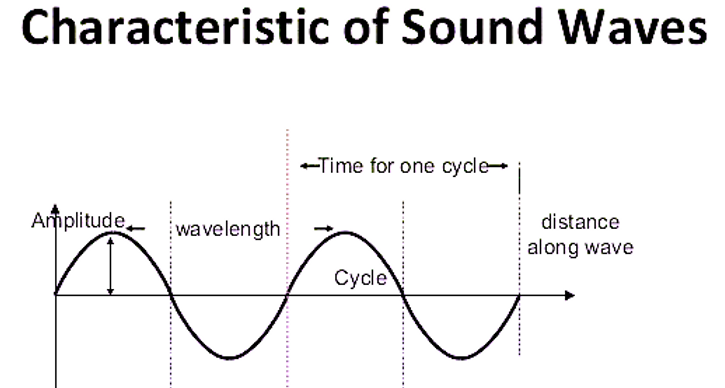Search

# Characteristics of Sound Waves

• Amplitude

The amplitude of a periodic variable is a measure of its change over a single period (such as time or spatial period). There are various definitions of amplitude (see below), which are all functions of the magnitude of the difference between the variable's extreme values. In older texts, the phase is sometimes called the amplitude.

• Wavelength

A wavelength is a measure of the distance between two identical peaks or crests high points or between two identical troughs low points in a wave.

• Time-Period

The time required to produce one complete wave or cycle or cycle is called time-period of the wave. Now, one complete wave is produced by one full vibration of the vibrating body. So, we can say that the time taken to complete one vibration is known as time-period. It is denoted by letter T. The unit of measurement of time-period is second (s).

• Frequency

The number of complete waves or cycles produced in one second is called frequency of the wave. Since one complete wave is produced by one full vibration of the vibrating body, so we can say that the number of vibrations per second is called frequency. For example: if 10 complete waves or vibrations are produced in one second then the frequency of the waves will be 10 hertz or 10 cycles per second. Do you know that the frequency of a wave is fixed and does not change even when it passes through different substances?

The S.I unit of frequency is hertz or Hz. A vibrating body emitting 1 wave per second is said to have a frequency of 1 hertz. That is 1 Hz is equal to 1 vibration per second.

Sometimes a bigger unit of frequency is known as kilohertz (kHz) that is 1 kHz = 1000 Hz. The frequency of a wave is denoted by the letter f.

The frequency of a wave is the same as the frequency of the vibrating body which produces the wave.

• Velocity or Speed

The distance travelled by a wave in one second is called the velocity of the wave or speed of the wave. It is represented by the letter v. The S.I unit for measuring the velocity is metres per second (m/s or ms-1).